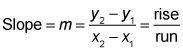##### Geometry For DummiesThe slope of a line on the coordinate plane basically tells you how steep the line is. If you know the rise and run of a line, you can calculate its slope using the slope formula.

Slope formula: The slope of a line containing two points, (x1, y1) and (x2, y2), is given by the following formula (a line's slope is often represented by the letter m):Note: It doesn't matter which points you designate as (x1, y1) and (x2, y2); the math works out the same either way. Just make sure that you plug your numbers into the right places in the formula.

The rise is the "up distance," and the run is the "across distance" shown in the above figure. To remember this, note that you rise up but you run across, and also that "rise" rhymes with "y's."

Compare the following list with the second figure, which shows you that the slope of a line increases as the line gets steeper and steeper:

• A horizontal line has no steepness at all, so its slope is zero. A good way to remember this is to think about driving on a horizontal, flat road—the road has zero steepness or slope.
• A slightly inclined line might have a slope of, say, 1/5.
• A line at a 45-degree angle has a slope of 1.
• A steeper line could have a slope of 5.
• A vertical line (the steepest line of all) sort of has an infinite slope, but math people say that its slope is undefined. (It's undefined because with a vertical line, you don't go across at all, and thus the run in

would be zero, and you can't divide by zero). Think about driving up a vertical road: You can't do it—it's impossible. And it's impossible to compute the slope of a vertical line.

The lines you see in the second figure have positive slopes (except for the horizontal and vertical lines). So what about lines with negative slopes? Actually, there are a couple of ways to distinguish the two types of slopes:
• Lines that go up to the right have a positive slope. Going from left to right, lines with positive slopes go uphill.
• Lines that go down to the right have a negative slope. Going from left to right, lines with negative slopes go downhill.